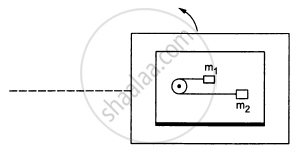Share

# A Table with Smooth Horizontal Surface is Placed in a Circle of a Large Radius R. a Smooth Pulley of Small Radius is Fastened to the Table. - Physics

ConceptCircular Motion

#### Question

A table with smooth horizontal surface is placed in a circle of a large radius R (In the following figure). A smooth pulley of small radius is fastened to the table. Two masses m and 2m placed on the table are connected through a string going over the pulley. Initially the masses are held by a person with the string along the outward radius and then the  system is released from rest (with respect to the cabin). Find the magnitude of the initial acceleration of the masses as seen from the cabin and the tension in the string.#### Solution

Let the bigger mass accelerates towards left with acceleration a.
Let T be the tension in the string and ω be the angular velocity of the table.
From the free body diagram, we have :

$T - ma - m \omega^2 R = 0 . . . (i)$

$T + 2ma - 2m \omega^2 R = 0 . . . (ii)$

$\text { On subtracting } eq . (i) \text { by eq .}(ii), \text { we get : }$

$3ma = m \omega^2 R$

$\Rightarrow a = \frac{\omega^2 R}{3}$

Substituting the value of a in eq. (i), we get :

$T = m\left( \frac{\omega^2 R}{3} \right) + m \omega^2 R$

$\Rightarrow T = \frac{4}{3}m \omega^2 R$

Is there an error in this question or solution?

#### APPEARS IN

Solution A Table with Smooth Horizontal Surface is Placed in a Circle of a Large Radius R. a Smooth Pulley of Small Radius is Fastened to the Table. Concept: Circular Motion.
S